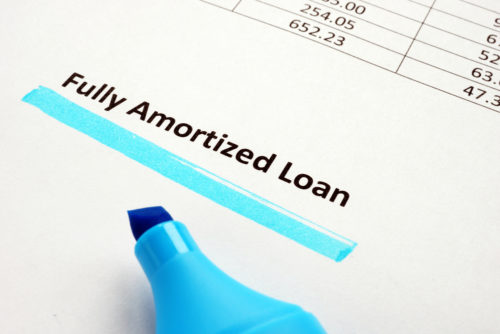# What Is Car Loan Interest and How Do I Calculate it?To understand a car loan’s interest rate, you must first understand what interest is. Any time you take out a loan, you have to pay back the amount you borrowed with interest. Interest is a fee that the financial institution you borrow from charges for using their money to buy big-ticket items such as cars.

When it comes to your car loan interest rate, the most commonly used kind of interest is simple interest. Initially, the majority of your monthly payments go towards interest. As time goes on, a shift occurs so that by the end of the loan, your monthly payment goes towards the principal balance. Wondering how to calculate interest on your car loan or even how interest works? Read on to learn more about car loan interest and how to calculate it.

## What Is Loan Amortization?

Amortization, meaning the process of paying your loan over time, describes how simple interest rate car loans are paid back. Amortizing a loan means that more of your payment is applied to your interest at the start of the loan when your principal balance is highest.

As the principal amount lessens, the amount you pay towards interest does, too, and you end up paying more toward your principal. Most loans, such as car loans, student loans, and mortgages use amortization to ensure the loan gets paid off in a certain amount of time.

## How to Calculate Car Loan Interest

Discover some of the easiest ways to calculate the interest on your car loans.

### Interest Rate Formula

Wondering how to find interest rate information for your simple interest car loan? You can calculate it manually. A=P(1+rt) is the original formula for calculating interest, where:

• A is the amount you owe (your principal plus interest).
• P is the principal, which is the amount you borrowed.
• r is the annual rate of interest expressed as a decimal (R/100).
• R is the annual rate of interest expressed as a percent (r*100).
• t is the time period of the loan.

Understanding how to calculate interest manually can help you determine which auto loan is right for you. Let’s say the principal amount (P) of your loan is \$10,000 and your rate of interest is 3.5%. First, convert the percentage, R, to a decimal, r. In this case, that would be 0.035 per year. Then plug all of that information into the equation, including the time frame you have to pay off your loan. It’s best to use years in this case.

Let’s say you have five years to pay off your \$10,000 car loan, which you received at an interest rate of 3.5%. You can calculate how much you’ll pay in interest by using the interest rate formula, as demonstrated below.

\$11,750.00 = \$10,000(1 + (0.035 × 5))

The total amount accrued (A), principal (\$10,000) plus interest (a rate of 3.5% for five years), is \$11,750. To figure out how much you’re paying in interest, subtract your principal from your accrued amount. In this case, it means that you’re paying \$1,750 in interest.

## What Affects Interest?

Before you take out a loan, it’s important to understand the factors that could affect your interest rate. This information should be available to you from the lender prior to agreeing to the loan’s terms and conditions. Oftentimes, you can also find this information online. Familiarize yourself with what can cause your interest rate to increase, including your principal payment, the term of your loan, a low down payment, a variable interest rate, and your overall credit score.

### Principal Payment

The principal, or the amount of money you borrow, is one of the factors that affects interest. Realistically, you should only borrow what you know you can pay back with the addition of interest. Because lenders want compensation for giving you money, the interest they charge helps pay for the lender’s services when you purchase a car.

### Loan Term

The term of your car loan can also impact interest. Shorter loan terms generally mean higher repayments but less interest in the long run. Conversely, longer terms allow for lower monthly repayments but can cost more in interest over the life of the car loan. It comes down to what your budget can afford.

### Down Payments

Down payments take away from the principal amount borrowed. This means the more money you put towards a down payment, the more you can save in interest over time. If you can afford to, make a large down payment towards your principal balance to keep what you pay in interest at a minimum.

### Interest Rates

Interest rates can change yearly or even monthly. Be sure to analyze the interest rates each financial institution offers before deciding where to take out an auto loan. Remember to use the basic annual interest rate when calculating interest. The comparison rate takes into account any fees and will give you the true value of the loan.

### Credit Scores

Your credit score, which determines how likely you are to pay back a loan, can drastically impact your interest rate. A higher credit score lets financial institutions know you’re responsible and will repay your loan so that they feel comfortable giving you a lower interest rate. Conversely, someone with a poor credit score will receive a higher interest rate so that the bank can protect themselves should they default on their repayment.

Image Source

## Related Posts

### Credit Card Utilization Calculator: How to Calculate and How It Impacts Your Credit Score